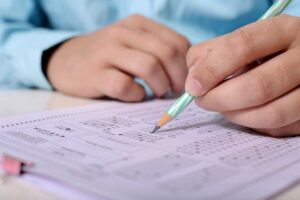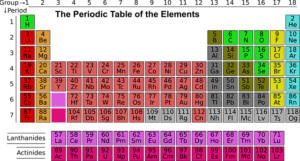# Blog## Format of O Level Additional Mathematics (syllabus 4049)

The Singapore O Level Additional Mathematics (also called A- Math or Add- Math) consists of 2 papers. Any of the topics in the Add Math…## What’s tested in O Level Additional Mathematics (syllabus 4049)

Do you know what’s tested in O Level Additional Mathematics? Well, the easiest way to find out is to look at your textbook. The latest…## Adapting to solve this almost linear simultaneous equation for O Level Add Math

Simultaneous equations are often a part of a question in O level Additional Math (also called A Math or Add Maths in short). You can…## Solving Simultaneous Equations – O Level Additional Mathematics

Get our simultaneous equations free course based on the Singapore additional Math syllabus right now! This course consists of 3 video lectures and 1 worksheet…## Periodic Table for O Level Chemistry

Periodic Table O- Level Chemistry is what I call a buffer topic in O Level Chemistry. It is a relatively easy-to-understand topic and there isn’t…